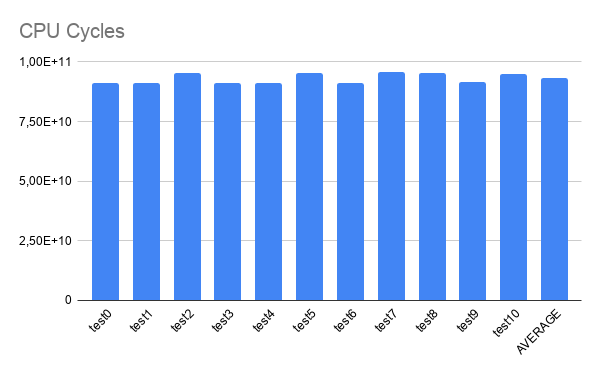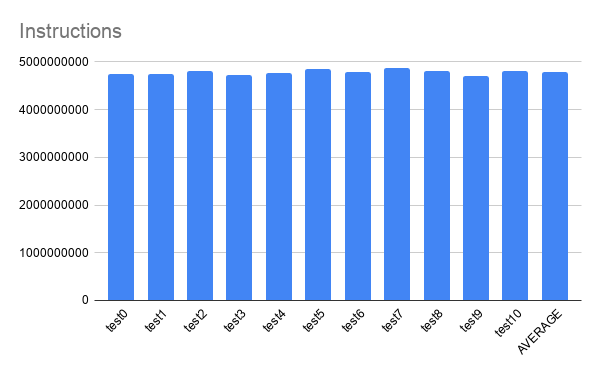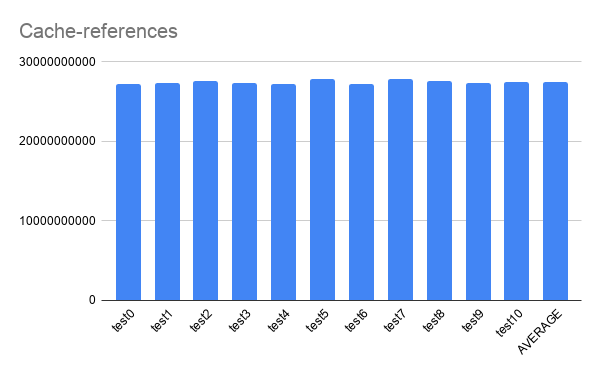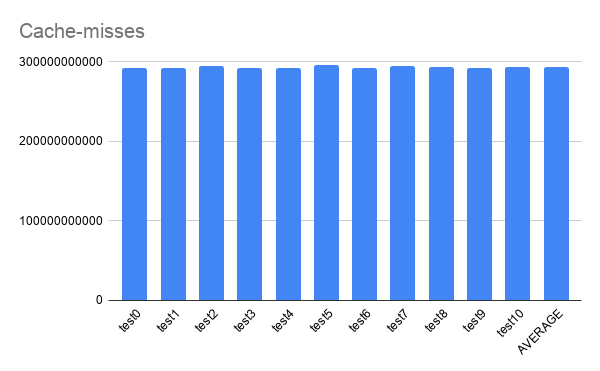# AI Profiling for POWER

Although there is plenty information on AI profiling for x86_64 and ARM architectures, there is almost none on POWER.

With that motivation in mind, this post aim to share some results on this subject.

The program profiled was a python script that had a pre-trained ResNet50 with ImageNet weights, which was obtained from TensorFlow API.
The profiling was done using Perf for collecting PMU data and ipmitool for energy consumption data.

Requirements for the pyhton script:

• Bare-metal machine
• ipmitool
• python 3.6
• TensorFlow 2.1.1

Machine Stats:

• POWER9 Processor
• CPU(s): 128
• On-line CPU(s) list: 0-127
• Core(s) per socket: 16
• Socket(s): 2
• NUMA node(s): 2
• Model: 2.2

You can find information on how to install TensorFlow on POWER in this post: https://openpower.ic.unicamp.br/post/building-tensorflow-on-power/

Eleven tests were executed.

``````from tensorflow.keras.applications.resnet50 import ResNet50
from tensorflow.keras.preprocessing import image
from tensorflow.keras.applications.resnet50 import preprocess_input, decode_predictions
import numpy as np
import sys
import time
from datetime import datetime

begin = time.time()

#folder = sys.argv
length = 731
length = int(sys.argv)
if (length > 731):
print("Using maximum length: 731")
lenght = 731
again = int(sys.argv)
folder_name = "hot_dog"

model = ResNet50(weights='imagenet')

images = []
count = 0
for i in range(length):
img_path = folder_name + '/' + str(i) + '.jpg'
images.append(image.img_to_array(img))
images[i] = np.expand_dims(images[i], axis=0)
images[i] = preprocess_input(images[i])

for j in range(again):
for i in range(length):
prediction = model.predict(images[i])
#print(decode_predictions(prediction[i], top=1))
strPrediction = decode_predictions(prediction, top=1)
if (strPrediction == 'hotdog'):
count += 1
else:
#print(str(i) + " -> " + strPrediction)
pass

print("Begin: " + datetime.utcnow().strftime("%H:%M:%S"))
print("End: " + datetime.utcnow().strftime("%H:%M:%S"))
print("RIGHTS: {}".format(count))
print("WRONGS: {}".format(again*length - count))
print("ACC: {}".format(count/(again*length)))
print("Time Elapsed: {}s".format(time.time() - begin))
``````

Because the model is pre-trained, it obtained the same classification accuracy for every test.

``````RIGHTS: 433
WRONGS: 67
``````

### Profiling using perf.

Perf is a profiling program included with the Linux kernel. Here it was used to instrument CPU performance counters.

PMUs used:

``````branches,
branch-misses,
cache-misses,
cache-references,
cycles,
instructions,
idle-cycles-backend,
idle-cycles-frontend.
``````

The following graphs shows the data fetched from those PMUs.
Graphs:
CPU Cycles:Instructions:Cache-references:Cache-misses:Branches:Branch-misses:Time Elapsed:### Energy consumption.

Make sure you are running on a bare-metal machine.

How to use the ipmitool to get power consumption data: Install ipmitool through:

``````sudo apt-get install ipmitool
``````

Then run the command:

``````sudo ipmitool dcmi power reading
``````

Which is going to give you the output:

``````
Minimum during sampling period:                248 Watts
Maximum during sampling period:                263 Watts
Average power reading over sample period:      257 Watts
IPMI timestamp:                           Sun Nov  8 19:51:18 2020
Sampling period:                          00000005 Seconds.

``````

This command was executed continuosly for 1500 seconds using a python script that would parse the results into a csv file. The data was used to plot the following graph:It is possible to see the average of energy consumption for each test and, at the end, the energy consumption going back to a normal state. It can also be observed that there is an increase close to 100W when a test begins to run.